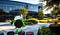# LeetCode Two Sum -JavaScript brute-force and optimized;

There are thousands of ways to solve “simple” problems in leet-code; today im going to go over a “brute-force” solution; and after explain the not so intuitive approach, and hopefully give YOU a better understanding how the “not so intuitive” approach works.

‘The ability to speak exactly is intimately related to the ability to know exactly.” — Wendell Berry

Big shout-out to jakesully at leetcode.com/jakesully for coming up with an awesome solution;Awesome picture of Google. Not really relevant, might be the reason you clicked on this story.

So, lets get started.

Given an array of integers, return indices of the two numbers such that they add up to a specific target.

You may assume that each input would have exactly one solution, and you may not use the same element twice.

So, what does this tell us? We have an array of integers,and IF two of the elements in our set will equal out to our target value we need to RETURN the indexes corresponding to those numbers.

`pseudo-code for Brute Force //so out input will be an array of numbers [0,3,5,2,1,4] and our target will be a number between -infinity and +infinity//we need to add two of our numbers together to reach the target// we can do with through looping in a forloop, iterating over the entire array.//because we need to add two numbers, we can use a nested for-loop//if arr[index1] + arr[index2] = targetNumber, we need to return[index1, index 2]function(array, targetNumber){//check length to make sure it is not less than or equalto 1        if(array.length <=1)return null;//we will create two loops nested        for(let i = 0; i < array.length; i++){// we will start our second index at index1 + 1            for(let j = i+1; j < array.length; j++){//and check if the array values at each index equal the targetNumber                if(array[i]+array[j] === target){                    return [i,j]            }         }}`

If you took time to read through the pseudo-code you can see the simplicity in the solution, essentially all we need to do is run through our array a bunch of times until our values match up to equal the target value, at that point we return the value.

But, this is extremely inefficient, its really gross, but its a good starting point.

We will need to loop through our array, there’s no getting around it(if there is a way, please comment below), but, can you think of a way that we would only have to run through it once?

What if we determine what numbers we need to look for by knowing what we already have?

So we are going through this array, if we know what number we are looking for and we know a number that we have, for example;

`We have an array of [1,2,3,4,5] and we need to find a pair that equal 8; if we have a 1, we will need a 7, if we have a 2, we will need a 6...if we have a 3, we will need a 5. numberWeWant - numberWeHave = THE NUMBER WE NEED ::party::party::`

So we need to figure out a way to keep track of the numbers we have and the number we need; this can be done with an object and a little bit of ingenuity ;

WARNING!!: This code may throw you off if you’re not a mathMagician… I’m funny, I know….

• 1x Function
• 1x Object instantiated
• 1x For-Loop
• 1x If Logic
• 1x Object-Builder
• 1x Return-er

So,

If looks simple enough, and it really is.